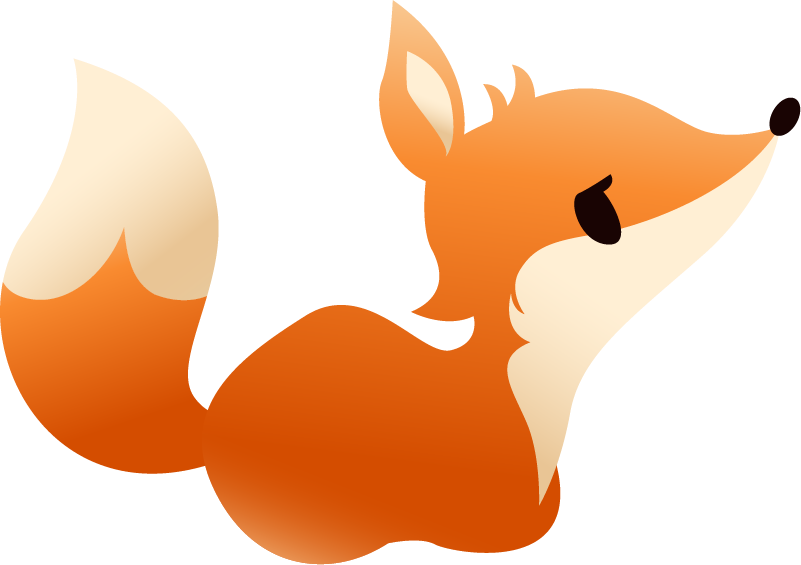# "Batch size" feature doesn't work?

So when I go to my deck and change the batch size, it does nothing. Items per lesson = lesson size. I thought batch size was supposed to equal the number of items within a set/lesson, i.e. if batch size = 10 and lessons per day = 3 you’d get 30 cards a day.

Am I missing something? The only way I can study 30 cards a day is if I increase the lesson number to 30.

1 Like

Batch size is used to determine how many lessons you get before you go to the quiz part of the lessons. So having 30 lessons per day and a batch size of 5 would work like this:

30 lessons -> learn 5 cards -> quiz about those 5 cards -> learn 5 cards -> quiz about those 5 cards -> repeat until done with all 30 cards.

3 Likes

Oh, that makes sense. I didn’t really understand how it worked. Thank you very much!

1 Like

No problem at all, let me know if you have any other questions